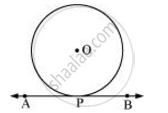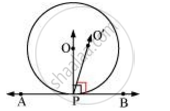# Prove that the perpendicular at the point of contact to the tangent to a circle passes through the centre - Mathematics

Prove that the perpendicular at the point of contact to the tangent to a circle passes through the centre

Prove that the perpendicular at the point of contact to a circle passes through the centre of the circle.

#### Solution

Let us consider a circle with centre O. Let AB be a tangent which touches the circle at P.We have to prove that the line perpendicular to AB at P passes through centre O. We shall prove this by contradiction method.

Let us assume that the perpendicular to AB at P does not pass through centre O. Let it pass through another point O’. Join OP and O’P.As perpendicular to AB at P passes through O’, therefore,

∠O’PB = 90° … (1)

O is the centre of the circle and P is the point of contact. We know the line joining the centre and the point of contact to the tangent of the circle are perpendicular to each other.

∴ ∠OPB = 90° … (2)

Comparing equations (1) and (2), we obtain

∠O’PB = ∠OPB … (3)

From the figure, it can be observed that,

∠O’PB < ∠OPB … (4)

Therefore, ∠O’PB = ∠OPB is not possible. It is only possible, when the line O’P coincides with OP.

Therefore, the perpendicular to AB through P passes through centre O

Concept: Number of Tangents from a Point on a Circle
Is there an error in this question or solution?
Chapter 10: Circles - Exercise 10.2 [Page 214]

#### APPEARS IN

NCERT Class 10 Maths
Chapter 10 Circles
Exercise 10.2 | Q 5 | Page 214
RD Sharma Class 10 Maths
Chapter 8 Circles
Exercise 8.2 | Q 22 | Page 35

Share